# Fall Detection and Motion Analysis Using Visual Approaches

Title: Fall Detection and Motion Analysis Using Visual Approaches
Xin Lin Lau , Tee Connie, Michael Kah Ong Goh, Siong Hoe Lau

Corresponding email:

Lau, X.L., Connie, T., Goh, M.K.O., Lau, S.H., 2022. Fall Detection and Motion Analysis Using Visual Approaches. International Journal of Technology. Volume 13(6), pp. 1173-1182

326
 Xin Lin Lau Faculty of Information Science & Technology, Multimedia University, 75450, Melaka, Malaysia Tee Connie Faculty of Information Science & Technology, Multimedia University, 75450, Melaka, Malaysia Michael Kah Ong Goh Faculty of Information Science & Technology, Multimedia University, 75450, Melaka, Malaysia Siong Hoe Lau Faculty of Information Science & Technology, Multimedia University, 75450, Melaka, Malaysia
Email to Corresponding Author

Abstract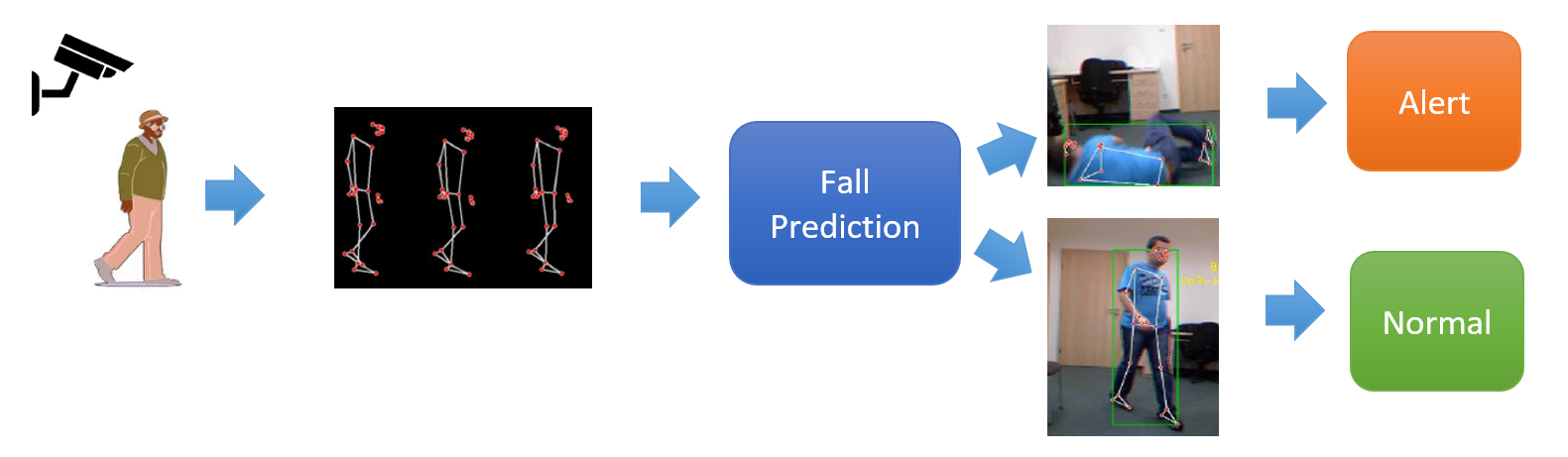Falls are considered one of the most ubiquitous problems leading to morbidity and disability in the elderly. This paper presents a vision-based approach toward the care and rehabilitation of the elderly by examining the important body symmetry features in falls and activities of daily living (ADL). The proposed method carries out human skeleton estimation and detection on image datasets for feature extraction to predict falls and to analyze gait motion. The extracted skeletal information is further evaluated and analyzed for the fall risk factors in order to predict a fall event. Four critical risk factors are found to be highly correlated to falls, including 2D motion (gait speed), gait pose, 3D trunk angle or body orientation, and body shape (width-to-height ratio). Different variants of deep architectures, including 1D Convolutional Neural Network (CNN), Long Short-Term Memory (LSTM) Network, Gated Recurrent Units (GRU) model, and attention-based mechanism, are investigated with several fusion techniques to predict the fall based on human body balance study. A given test gait sequence will be classified into one of the three phases: non-fall, pre-impact fall, and fall. With the attention-based GRU architecture, an accuracy of 96.2% can be achieved for predicting a falling event.

Attention mechanism; Deep learning; Fall detection; Gated Recurrent Unit (GRU); Vision approach

Introduction

Falls have been one of the predominant threats to people’s health, particularly in aged citizens. Falls in the elderly are recognized as one of the leading public health concerns because of the high prevalence, severe consequences and significant weight on society. Fall detection has been extensively studied in many research papers. Different from the other studies, the primary focus of this study is to analyzevision-based data that may contribute to identifying specific behaviors that are considered as precursors to falls and whether a subject has an increased risk of falling (Yu et al., 2020).

Generally, there are three types of fall detection approaches, including handheld-device-based, ambient-detector-based, and vision-based. In an ambient-detector-based approach, the subjects are required to wear the sensor device, and the signals data recorded will be further analyzed and classified for a fall. Compared to the vision-based approach, which only relies on a single camera, the sensor-based approach appears to be inconvenient and requires a higher cost. Hence, a visual-based approach is presented in this study as it provides unobtrusive monitoring from a far distance without human intervention. Besides, different deep neural networks that have similar spatiotemporal features are implemented and discussed to compare the performances in multivariate time-series data.
In this paper, skeleton data extracted using the MediaPipe Pose estimator are used for motion monitoring. We found that features including human pose, vertical trunk angle between hip and shoulder, human gait speed, and width-height ratio of human body rectangular are useful for detecting a fall event. These features are computed and classified and eventually divide the falling process into three categories: non-fall, pre-impact fall, and fall. Pre-impact fall is the process of the occurrence of a fall before the body collides with the floor, while fall is the event where the body inadvertently hits the ground. Four types of deep neural networks, namely LSTM, GRU, CNN, and Attention mechanism, are investigated in this study to predict a fall based on the human body balance study. In addition, different fusion techniques, including raw-level fusion, model stacking, and score-level fusion are tested. Overall, the proposed study could be denoted as a home-based solution for a safety mechanism that may substitute the need for costly rehabilitation (Forbes et al., 2020).

Experimental Methods

In this study, three public datasets, namely the Fall Dataset (Adhikari et al., 2017), UR Dataset (Kwolek & Kepski, 2014), and Multiple Cameras Fall Dataset (Auvinet et al., 2010) are used. The video sequences are pre-processed using the MediaPipe Pose pose estimator to retrieve 2D and 3D skeleton joint points, respectively. This is followed by developing a deep neural network with an attention-based mechanism to predict a fall based on a human body balance study and eventually divides the falling process into three categories: non-fall, pre-impact fall, and fall.

2.1. Human Key Point Extraction

Human key point detection is a process of detecting humans and localizing their skeleton key points. It is one of the crucial pre-processing methods to extract the interest points or key objects part of a human. These key points are spatial locations that represent the important features in an image. For example, the key parts include the nose, shoulder, elbow, hip, eye, knee, and ankle positions of the detected human. Key points are detected and extracted from movement realized by the subject with time-synchronization via MediaPipe Pose. These detected key points are then further computed to derive useful features to estimate if the subject in the video portrays a fallingbehavior. In this study, both the 2D and 3D holistic skeleton joints are extracted (Figure 1).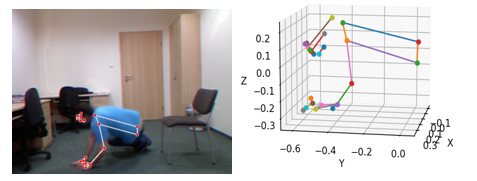Figure 1 Example of 3D MediaPipe Pose Detection

In the end, MediaPipe Pose returns results that contain 2D and 3D key point coordinates. In this paper, the 2D skeleton points are used for gait speed, skeleton posture points and width-height rectangular body ratio calculation while the 3D key points are used for trunk angle computation to predict a fall event. The 3D key points are an array with 33 key point objects, while each object has x, y, and z coordinates that are transformed into meter units. Each axis key points are normalized, which range from -1 to 1, and the origin of the 3D space starts from the hip center (0, 0, 0).

2.2. Fall Measurement

Gait and balance are major factors that contribute to falls as they are associated with morbidity and mortality. Therefore, evaluation of gait and balance is a vital step for identifying whether a person has an increased risk of falling.

2.2.1. Trunk Angle

The trunk angle is the angle formed by the trunk and the vertical plane traveling through the body's center of mass (hip). Trunk control is an important feature in the balance assessment of functional walking gait as it shows the ability of the trunk muscles to uphold a vertical or neutral position, withstand body weight and move selectively to keep the center of gravity over the support base. In other words, the trunk angle has a significant relation to trunk balance during functional activities and contribution to the falling probability (Karthikbabu et al., 2011).

Commonly, when the individual is standing, the body symmetry will be upright, and the angle of the vertical line through the centre of gravity will be at approximately 0 degrees to the shoulder position; when the individual is walking, the angle value will be in between 45 degrees to 90 degrees; when the individual is falling, the angle will be greater than 90 degrees.

To begin the calculation of trunk angle, the 3D key points acquired from MediaPipe Pose are used to compute the parameter set. Due to random ADL activities performed by the volunteers, there are three possibilities of trunk angle obtained from different views, such as side view, frontal view, or back view. These trunk angles may be generated from either the ?1 (x, y) axis, ?2 (x, z) axis, or ?3 (y, z) axis as shown in Figure 2b. Hence, three different trunk angles within one frame needed to be calculated, eventually selecting the most accurate trunk angle based on the optimized function.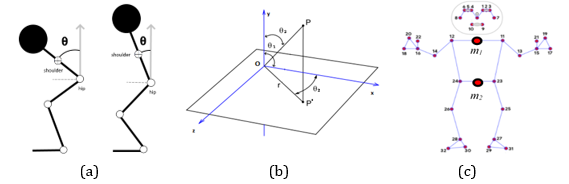Figure 2 Example of trunk angle: graphical skeleton joints (a, b), midpoint positions(c)

Firstly, the midpoint between the shoulder (landmark 11, 12), m1 (Equation 1), and midpoint between hips (landmark 23, 24), m2 (Equation 2) as shown in Figure 2c, are computed in the form of (Mx, My, Mz). These calculated midpoints are then passed to compute trunk angle.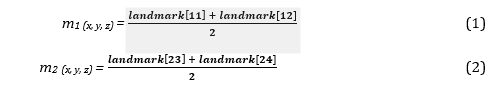To calculate the trunk angle, three points (shoulder midpoints, hip midpoints, and perpendicular endpoints) in the form of (i, j) are fed into the function. Next, the radian (Equation 3) and angle (Equation 4) for the particular joint are calculated. By using the arctan 2 function, vector 2 (V2) is calculated by subtracting the (Mi, Mj) point from the shoulder midpoint with (Hi, Hj) point from the hip midpoint, while vector 1 (V1) is calculated by subtracting (Ei, 0) point from the perpendicular endpoint with (Hi, Hj) point from the hip midpoint. The j coordinate for the perpendicular endpoint is always constant with the value of 0 (Ei, 0) as the angle is counted from the upright position. Moreover, angle inspection is also performed to avoid obtaining an invalid trunk angle that exceeds 180 degrees.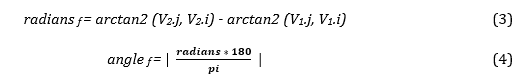To select the most accurate trunk angle from the three calculated trunk angles, the x-axis distance, d (Equation 5), between m1 and m2 is calculated by subtracting the x-coordinate values from m1 to m2. Next, the absolute value of the calculated distance (d) is obtained to assure the positive distance value.

d f = | m1.x – m2.x |                                                              (5)

In the case where the distance (d) is smaller than 15 units, the least trunk angle is selected because the short distance may indicate that the person may be facing front and the body symmetry may be in an upright position, thus, the trunk angle selected should be small. Conversely, in the case where the distance (d) is greater than or equal to 15 units, the largest trunk angle is selected because the large distance may indicate that the person may be facing from the side, and the shape of body symmetry may be bending;thus the trunk angle selected should be large.

2.2.2. Human Gait Speed

Gait speed is a fast, low-cost, dependable measure of fall analytical assessment for major health-related fields (Peel et al., 2013). Research has shown that there is a close association between gait speed and falls, whereby a single gait speed factor is sufficient to identify whether an individual is at a high risk of falling.

To calculate the gait speed, two important parameters are required, namely distance (D) and time per frame (T). Due to the different frame times within different video datasets, the frame times are calculated specifically for the three datasets. To obtain a single frame time (Fps) of a video in the dataset, all frames are extracted and calculated from the video. The number of frames extracted is divided by the total video time to get frames per second (Fps) (Equation 6). Next, the time of a single frame (T) is obtained by dividing 1 over frame per second (Fps).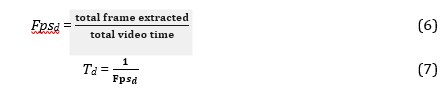Based on Equation 7, the time (Td) for a single frame in dataset 1, dataset 2, and dataset 3 are calculated as T1 = 0.033s, T2 = 0.066s, and T3 = 0.017s respectively. Apart from calculating time per frame (Td), the walking distance of every 12 continuous frames is accumulated and computed. In the first frame (fn), the midpoint coordinate between two feet (Mf(n)), Equation 8, is calculated and stored in a list.  After 12 continuous frames (fn+12), the midpoint coordinate of two feet (Mf(n+12)), Equation 9, is calculated, followed by subtracting with the midpoint (Mf(n)) of the first frame. The absolute value of the subtraction is obtained by means of distance (Df), Equation 10.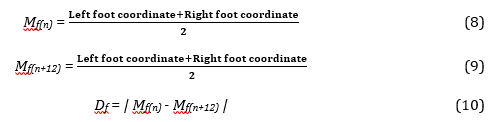After obtaining the walking distance in 12 continuous frames, the gait speed (Vf) within 12 frames is calculated using Equation (11), where Df is the distance within 12 frames, Td is the time per frame of the dataset.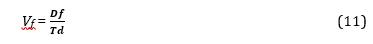2.2.3. Width-Height Ratio of Human Body Rectangular

The geometric parameters of the shape feature can be beneficial for predicting a fall because when a fall is detected, the contours of the body have a very obvious change in the feature shape (Chen et al., 2020).

Commonly, when the individual is standing or walking, the body symmetry may be presented in an upright position with a definite vertical rectangular shape of the external human bounding box; when the individual is sitting, the upper body symmetry may be bending, and the external human bounding box may tend to be in a square shape; when the individual has the risk of falling, the individual may have a supine position which will lead to a horizontal rectangle shape. Therefore, there are significant manifestation changes in the width–height body ratio when the individual is moving.

In this project, the ratio of width to the height of the outer human body rectangular (Rf) is calculated as Equation 12: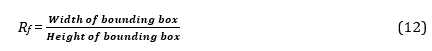In the case if the ratio is smaller than 1, the individual is considered to have the risk of falling; the shape of the presented bounding box is either square or a horizontal rectangle; the person is in a supine position. Conversely, in the case if the ratio is greater than or equal to 1, the individual is not considered to have the risk of falling; the shape of the presented bounding box is a vertical rectangle; the person is in an upright position (Figure 3).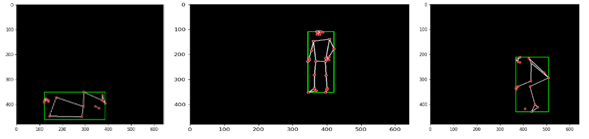Figure 3 Example of human body external rectangular bounding box

2.2.4. Skeleton Point

For skeleton points, to reduce computational time and increase prediction accuracy, only 13 vital normalized skeleton points that are highly correlated to falling behavior are extracted, including the skeleton positions of the head, left and right shoulder, ankle, hand, hip, knee, and feet. The X- and Y-axis of the chosen skeleton points are then further flattened into a vector (Equation 13) with a size of 26.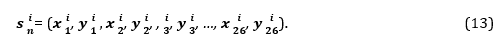2.3. Deep Learning Models

Generally, deep learning networks have a superior capability to symbolize high-level features, examine complex mappings from the input, and support multiple outputs (Fagbola et al., 2019; Berawi, 2020; Siswanto, 2022). In this section, different deep convolutional, recurrent neural networks, and data fusion techniques are applied for multimodality input data fusion to enhance the accuracy of falling prediction.

2.3.1. 1D Convolutional Neural Network (CNN)

Convolutional Neural Network (CNN) is a supervised, feed-forward, deep neural network model, commonly used for image processing and classification (MIAP, 2019). In this study, 1D-CNN is selected to process the gait features due to its ability to handle vector-based data. In Conv1D, 2D matrices are used for both kernels and feature maps, whereby the kernel is sliding along one dimension with an input dimension of 2.

In 1D CNN architecture configuration, 29 parallel feature maps with a kernel size of 2 are set, followed by a max-pooling layer with the same padding, then 64 parallel feature maps with kernel size of 2. To prevent overfitting on the training data, a dropout layer of 0.5 is added after the 1D CNN layers, then followed by flattening the learned features into a long vector, eventually passing it to a fully connected layer before the Softmax output layer to classify the final learned features into 3 categories.

2.3.2. Long Short-Term Memory (LSTM)

Long Short-Term Memory (LSTM) is a kind of recurrent neural network that is able to solve short or long -term dependency issues and vanishing gradientproblems. LSTM network is great at modeling sequential data as it uses a series of ‘gates’ to regulate the coming and leaving information in the network.

To start LSTM network training, a single LSTM hidden layer is defined with 128 memory units, and the return sequence is set to True to output all the hidden states of each time step. It is followed by a 0.5 dropout layer to reduce the overfitting issue during model training. Then, learned features are flattened into a long vector, eventually passing it to a fully connected layer before the Softmax output layer to classify the final learned features into 3 categories.

2.3.3. Gated Recurrent Unit (GRU) Model

Gated recurrent units (GRUs) are a gating mechanism that works similarly to LSTM, except that the cell state is removed in GRU. GRU has only two gates: reset gate and update gate. To decide the passing of information within the network, the two stated gates and hidden states are used. Due to lesser gates and training parameters used, GRU is comparatively less complex, executing and training faster than LSTM.

To start GRU network training, a single GRU hidden layer is defined with 128 memory units, and the return sequence is set to True to return the last output in the time steps. It is followed by a 0.5 dropout layer to reduce the overfitting issue during model training. Then, learned features are flattened into a long vector, eventually passing it to a fully connected layer before the Softmax output layer to classify the final learned features into 3 categories.

2.3.4. Attention Mechanism

The attention mechanism is introduced in this paper with the aim of enhancing the memorizing technique to a larger sequence of data while diminishing other parts. The attention mechanism is capable of dynamically emphasising a significant unit of the input sequence, eventually learning the connection between them.

To customise the Attention layer, three overriding methods are defined: init (), build(), and call(). In the build() method, when the input size is passed to the layer, weights, and bias are automatically tuned and added via the add_weights() method with ‘trainable’ set to True. In the call() method, the aligned scores, weights, and context are computed to map the inputs to outputs. The attention layer is added to the LSTM and GRU architectures. When defining the model, attention layer is added right after the first architecture layer, followed by adding other fully connected layers, for instance, the dropout and dense layer after it.

2.4. Fusion

In this paper, three different levels of fusions are investigated. The fusion approaches include 1st and 2nd raw-level fusion, model stacking, and score-level fusion. Model fusion is introduced in this paper to harvest a higher model performance that is capable of choosing the best features computed from skeleton points.

In the 1st Raw-Level Fusion, two inputs are constructed separately for the model training. The first input fuses the 3 features that have the same input size (1,35), including gait speed (1,35), trunk angle (1,35), and rectangular body ratio (1,35), while the second input size is a standalone input which consists of only flattened skeleton points (35,26). Due to the two inputs constructed, the networks are defined to accept two inputs with different dimensions. In this case, two branches are created in the network; the first branch accepts (3,35) input while the second branch accepts (35,26) input.

In 2nd raw-level fusion, only one input is constructed for the model training. The constructed input size is (35,29), which concatenated the raw data input of gait speed (1,35), trunk angle (1,35), rectangular body ratio (1,35), and flattened skeleton points (35,26). The raw data of the four features are then flattened and fused together into an input size of (35,29). In raw-level fusion experiments, several multi-input short-term load forecasting models using LSTM, GRU, attention-based LSTM, GRU, and CNN models are constructed for the aim of achieving performance improvements.

On the other hand, in model stacking, several different sub-networks are embedded or stacked into a greater multi-headed neural network. Several models from 1st and 2nd Raw-Level Fusion models are stacked together to discover different combinations of architectures to best combine the weights of the sub-networks cooperatively, improve the performance of the single models and harvest the best prediction result.

In score-level fusion, at first, a total of five models (LSTM + Attention-based LSTM + GRU + Attention-based GRU + CNN) from 1st raw-level fusion and 2nd raw-level fusion is appended into a ‘model’ list, respectively. Subsequently, the testing dataset is used to predict labels with the loaded models. The predicted labels are then saved in a ‘labels’ list for later label voting. In this study, the majority voting scheme is applied.

Results and Discussion

The proposed methods are tested on three public datasets: UR fall dataset (URFD), multiple cameras (Multicam), and Fall detection dataset (FDD). A total of 10,065 sequence datasets, consisting of 3202 sequences from the Fall dataset, 3537 sequences from the UF dataset, and 3330 sequences from the Multiple Cameras Fall Dataset are collected and split into 70% training set, 15% testing set, and 15% validation set for learning module.

In all the architectures, sparse categorical cross entropy is used as the loss function; the Adam optimizer is adopted. The correct recognition rate is used as the accuracy metric to judge the performance of the models. Besides, early stopping is also applied to stop the model training when the monitored metric has stopped improving.

Table 1 Table of Comparison between Experimental Results

 Model Accuracy Feature Input size LSTM LSTM (Attention) GRU GRU (Attention) CNN Standalone Feature Model Gait Speed (35,1) 71.08% 70.06% 71.32% 79.09% 63.20% Trunk Angle (35,1) 76.65% 70% 78.63% 84.41% 67.08% Body Ratio (35,1) 76.17% 71.35% 75.93% 75.86 % 72.97% Skeleton Point (35,26) 90.67% 83.84% 90.91% 88.53% 91.12% 1st Raw-Level Fusion Gait Speed, Trunk Angle, Body Ratio (3,35) 71.08% 70.06% 71.32% 79.09% 63.20% Skeleton Point (35,26) 2nd Raw-Level Fusion Gait Speed, Trunk Angle, Body Ratio, Skeleton Point (35,29) 95.72% 91.23% 95.17% 96.23% 93.83% Model Stacking on 1st Raw-Level Fusion LSTM 88.68% - 88.89% 91.42% 89.10% 91.48% LSTM (Attention) 84.71% 88.89% - 91.54% 61.96% 91.45% GRU 88.59% 91.42% 91.54% - 91.63% 91.6% GRU (Attention) 91.39% 89.10% 61.96% 91.63% - 91.54% CNN 90.45% 91.48% 91.45% 91.54% 91.6% - 5 models 91.57% Model Stacking on 2nd Raw-Level Fusion LSTM 95.72% - 95.66% 96.15% 95.69% 95.4% LSTM (Attention) 91.23% 95.66% - 96.08% 64.16% 96% GRU 95.17% 96.15% 96.08% - 96.18% 96.1% GRU (Attention) 96.23% 95.69% 64.16% 96.18% - 93.8% CNN 93.83% 95.4% 96% 96.1% 93.8% - 5 models 95.87% Score-Level Fusion 1st Raw-Level Fusion (3,35), (35,26) 90.34% 2nd Raw-Level Fusion (35,29) 96.14%

Based on the experimental results on three datasets presented in Table 1, model architectures in 2nd raw-level fusion tend to give slightly better performance when compared with the model architectures in standalone feature and 1st raw-level fusion. In 2nd raw-level fusion, the average accuracy rate for the five architectures falls at 94.4%, while in 1st raw-level fusion, the average accuracy rate for the five architectures falls at 88.9%.
The accuracy results show that 2nd raw-level fusion performed better than 1st raw-level fusion, although 2nd raw-level fusion has a slight overfitting. This might be due to the potential loss of correlation in the mixed 1st raw-level space as 1st raw-level fusion has two sub-models to be first concatenated before merging them into a meta-model. Therefore, it requires more complex computation for every modality due to the separate supervised learning stages. On the other hand, the appropriate fusion of the raw data results in 2nd raw-level fusion, exploiting the cross-correlations between the data features, eventually giving the chance to improve the ideal performance of the network. Therefore, we can conclude that the unimodal performance (one flattened input) is superior to the multimodal (multiple inputs) performance, 96.23% (Attention-based GRU) from 2nd raw-level fusion and 91.3% (Attention-based GRU) from 1st raw-level fusion.

By comparing Attention-based architectures with normal architectures, it is shown that most of the Attention-based architectures outperformed the normal architectures. By implementing an Attention mechanism in the architecture, it allows the decoder to focus exclusively on certain areas of the input; eventually, the architecture is able to pay close attention to all of the inputs that is significant in generating the output, producing better performance. In comparison to the LSTM and 1D CNN models, the GRU model performs better in terms of accuracy and learning curve since it can learn, accept longer periods with minimal impact on the performance, and have a stable learning curve when the plot of training loss and validation loss diminishes. Thus, the end result demonstrates that GRU models are considered robust.
In model stacking, the average accuracy rate for the stacked architectures falls at 91.58% (1st raw-level fusion) and 95.85% (2nd raw-level fusion), while in score-level fusion, the majority voting accuracy that consists of five architectures (LSTM, LSTM-Attn, GRU, GRU-Attention, 1D CNN) in 1st and 2nd raw-level fusion architectures are 90.34% and 96.14% respectively. Based on the results, by implementing model stacking and score-level fusion, these models slightly outperform the baseline method on fall risk assessment when it combines the capabilities of the high-performing models from 1st and 2nd raw-level fusion. However, these stacked or majority-voted models are still not performing better than the single Attention-based GRU model from 2nd raw level fusion (96.23%). This might be due to the complex interpretation of the model assembling, which can cause the unseen underlying data patterns and are not able to be well trained, therefore, the accuracy results between the stacked models might be averaged or decreased in this case. To conclude, the Attention-based GRU model from 2nd raw-level fusion, however, still outperformed the others and achieved the highest accuracy prediction of 96.23% on the three datasets.

Conclusion

Vision-based approaches have been implemented and applied in gathering information from human daily living activities to evade the forgotten issue with portable detector devices and complicated installation problems in ambient-based devices. Therefore, the vision-based fall predictor presented in this paper is believed to have a strong step closer to real-world deployment. This research analyses different aspects of important body symmetry features that were found highly correlated to falls and could be portrayed as an early preventive intervention to avoid fall-related injuries before the body hits the ground.

Acknowledgement

This project is supported by the Fundamental Research Grant Scheme (Grant no. FRGS/1/2020/ICT02/MMU/02/5). The authors would also like to thank the providers of the Fall Dataset, UR Dataset, and Multiple Cameras Fall Dataset for sharing their databases.

Adhikari, K., Bouchachia, H., Nait-Charif, H., 2017. Activity Recognition for Indoor Fall Detection Using Convolutional Neural Network. In: 2017 Fifteenth IAPR International Conference on Machine Vision Applications (MVA), pp. 81–84

Auvinet, E., Rougier, C., Meunier, J., St-Arnaud, A., Rousseau, J., 2010. Multiple Cameras Fall Dataset. Technical Report 1350, DIRO - Université de Montréal, Canada

Berawi, M.A., 2020. Managing Artificial Intelligence Technology for Added Value. International Journal of Technology, Volume 11(1), pp. 1–4

Chen, W., Jiang, Z., Guo, H., Ni, X., 2020. Fall Detection Based on Key Points of Human-Skeleton Using OpenPose. Symmetry, Volume 12(5), p. 744

Fagbola, T.M., Thakur, C.S., Olugbara, O., 2019. News Article Classification using Kolmogorov Complexity Distance Measure and Artificial Neural Network. International Journal of Technology. Volume 10(4), pp. 710–720

Forbes, G., Massie, S., Craw, S., 2020. Fall Prediction Using Behavioural Modelling from Sensor Data in Smart Homes. Artificial Intelligence Review, Volume 53(2), pp. 1071–1091

Karthikbabu, S., John, M.S., Manikandan, N., Bhamini, K.R., Chakrapani, M., Nayak, A., 2011. Role of Trunk Rehabilitation on Trunk Control, Balance and Gait in Patients with Chronic Stroke: A Pre-Post Design. Neuroscience and Medicine, Volume 2(2), pp. 61–67

Kwolek, B., Kepski, M., 2014. Human Fall Detection on Embedded Platform Using Depth Maps and Wireless Accelerometer. Computer Methods and Programs in Biomedicine, Volume 117(3), pp. 489–501

MediaPipe Pose, (n.d.). MediaPipe. Available online at https://google.github.io /mediapipe/solutions/pose.html, Accessed on September 28, 2021

Peel, N.M., Kuys, S.S., Klein, K., 2013. Gait Speed as a Measure in Geriatric Assessment in Clinical Settings: A Systematic Review. The Journals of Gerontology: Series A, Volume 68(1), pp. 39–46

Siswanto, J., Suakanto, S., Andriani, M., Hardiyanti, M., Kusumasari, T.F., 2022. Interview Bot Development with Natural Language Processing and Machine Learning. International Journal of Technology, Volume 13(2), pp. 274–285

Yu, X., Qiu, H., Xiong, S., 2020. A Novel Hybrid Deep Neural Network to Predict Pre-impact Fall for Older People Based on Wearable Inertial Sensors. Frontiers in Bioengineering and Biotechnology, Volume 8, pp. 1–10